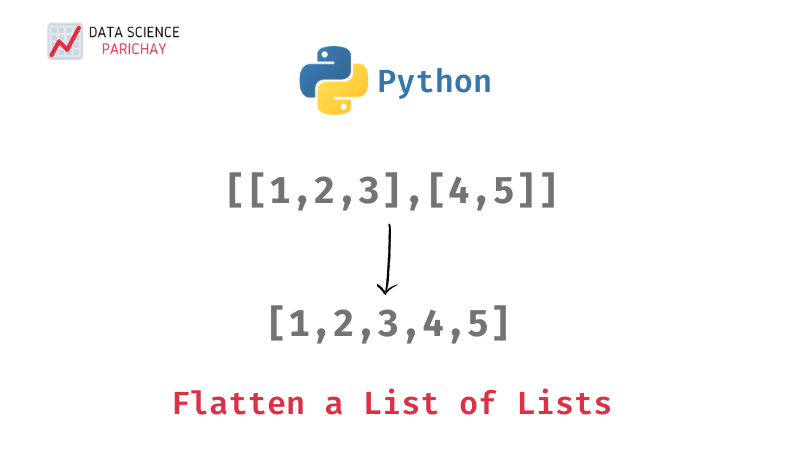# Python – Flatten a list of lists to a single list

In this tutorial, we’ll look at how to flatten a list of lists in Python to a single list through some examples. Suppose you have a list of lists –

``[['a','b','c'],['d','e','f'],['g','h','i']]``

Which you want to flatten out to a single list –

``['a','b','c','d','e','f','g','h','i']``

There are a number of ways to flatten a list of lists in python. You can use a list comprehension, the `itertools` library, or simply loop through the list of lists adding each item to a separate list, etc. Let’s see them in action through examples followed by a runtime assessment of each.

Here, we iterate through each item of all the subsequent lists in a nested loop and append them to a separate list.

``````# flatten list of lists
ls = [['a','b','c'],['d','e','f'],['g','h','i']]

# iterate through list of lists in a nested loop
flat_ls = []
for i in ls:
for j in i:
flat_ls.append(j)

# print
print("Original list:", ls)
print("Flattened list:", flat_ls)``````

Output:

``````Original list: [['a', 'b', 'c'], ['d', 'e', 'f'], ['g', 'h', 'i']]
Flattened list: ['a', 'b', 'c', 'd', 'e', 'f', 'g', 'h', 'i']``````

In the above example, the outer loop iterates over all the subsequent lists while the inner loop iterates over the items in each of the subsequent lists with each item being appended to the list `flat_ls`.

You can also use a list comprehension to basically do the same thing as in the previous step but with better readability and faster execution.

``````# flatten list of lists
ls = [['a','b','c'],['d','e','f'],['g','h','i']]

# using list comprehension
flat_ls = [item for sublist in ls for item in sublist]

# print
print("Original list:", ls)
print("Flattened list:", flat_ls)``````

Output:

📚 Data Science Programs By Skill Level

Introductory

Intermediate ⭐⭐⭐

🔎 Find Data Science Programs 👨‍💻 111,889 already enrolled

Disclaimer: Data Science Parichay is reader supported. When you purchase a course through a link on this site, we may earn a small commission at no additional cost to you. Earned commissions help support this website and its team of writers.

``````Original list: [['a', 'b', 'c'], ['d', 'e', 'f'], ['g', 'h', 'i']]
Flattened list: ['a', 'b', 'c', 'd', 'e', 'f', 'g', 'h', 'i']``````

Here, we use list comprehension to create a flattened list by keeping each item of each of the sublists in the original list of lists. Using list comprehension is similar to nested looping but has better readability (in this use case) and is faster (which we’ll see later in the article).

The python `itertools` standard library offers handy functionalities for working with iterables. Here, we break the list of lists into individual parts and then chain them together into a single iterable.

``````import itertools
# flatten list of lists
ls = [['a','b','c'],['d','e','f'],['g','h','i']]

# using itertools
flat_ls = list(itertools.chain(*ls))

# print
print("Original list:", ls)
print("Flattened list:", flat_ls)``````

Output:

``````Original list: [['a', 'b', 'c'], ['d', 'e', 'f'], ['g', 'h', 'i']]
Flattened list: ['a', 'b', 'c', 'd', 'e', 'f', 'g', 'h', 'i']``````

In the above example `itertools.chain()` function is used to join (or chain together) the iterables passed as parameters. Passing `*ls` to it results in the outer list to be unpacked as parameters which are then chained together. The following example illustrates this better.

``````ls1 = list(itertools.chain([1,2,3],[4,5],))
ls2 = list(itertools.chain(*[[1,2,3],[4,5],]))
print(ls1)
print(ls2)``````

Output:

``````[1, 2, 3, 4, 5, 6]
[1, 2, 3, 4, 5, 6]``````

You can see that for `ls1` we pass the iterables that we want to chain together as parameters to the `itertools.chain()` function. For `ls2`, since we’re passing a single list of lists, we need to unpack it to its component iterables using `*`.

Alternatively, if you’re not comfortable with using `*`, you can use the `itertools.chain.from_iterable()` function which doesn’t require you to unpack your list.

``````ls3 = list(itertools.chain.from_iterable([[1,2,3],[4,5],]))
print(ls3)``````

Output:

``[1, 2, 3, 4, 5, 6]``

Now let’s compare the different methods discussed above to flatten a list of lists for runtime.

``````%%timeit

ls = [['a','b','c'],['d','e','f'],['g','h','i']]
flat_ls = []
for i in ls:
for j in i:
flat_ls.append(j)``````
``1.45 µs ± 104 ns per loop (mean ± std. dev. of 7 runs, 1000000 loops each)``

``````%%timeit

ls = [['a','b','c'],['d','e','f'],['g','h','i']]
flat_ls = [item for sublist in ls for item in sublist]``````
``1.12 µs ± 128 ns per loop (mean ± std. dev. of 7 runs, 1000000 loops each)``

``````%%timeit

ls = [['a','b','c'],['d','e','f'],['g','h','i']]
flat_ls = list(itertools.chain(*ls))``````
``875 ns ± 20.2 ns per loop (mean ± std. dev. of 7 runs, 1000000 loops each)``

Of the three methods discussed, we found using `itertools.chain()` to be the fastest and using a direct nested loop to be the slowest. List comprehensions are not only fast but can be intuitive and readable for such cases (where they’re short and simple).

With this, we come to the end of this tutorial. We discussed some of the common ways of flattening a list of lists in python along with their examples and runtime performances. There are, however, other methods as well. The code examples and results presented in this tutorial have been implemented in a Jupyter Notebook with a python (version 3.8.3) kernel.

•